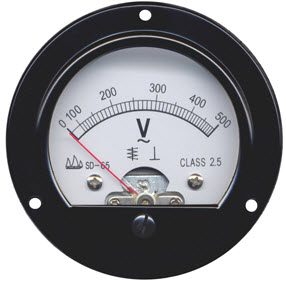Krishna
0

A voltmeter is an electronic instrument used to measure potential between any two points in an electric or electronic circuit in volts. A voltmeter can display readings in analog (a pointer across a scale in fraction of the voltage of the circuit) or digital (shows voltage directly as numerals) form. A voltmeter can also be used to measure AC, DC as well as RF currents.## Working Principle of Voltmeter

The main principle of voltmeter is that it must be connected in parallel in which we want to measure the voltage. Parallel connection is used because a voltmeter is constructed in such a way that it has a very high value of resistance. So if that high resistance is connected in series than the current flow will be almost zero which means the circuit has become open.

If it is connected in parallel, than the load impedance comes parallel with the high resistance of the voltmeter and hence the combination will give almost the same the impedance that the load had. Also in parallel circuit we know that the voltage is same so the voltage between the voltmeter and the load is almost same and hence voltmeter measures the voltage.

For an ideal voltmeter, we have the resistance is to be infinity and hence the current drawn to be zero so there will be no power loss in the instrument. But this is not achievable practically as we cannot have a material which has infinite resistance.